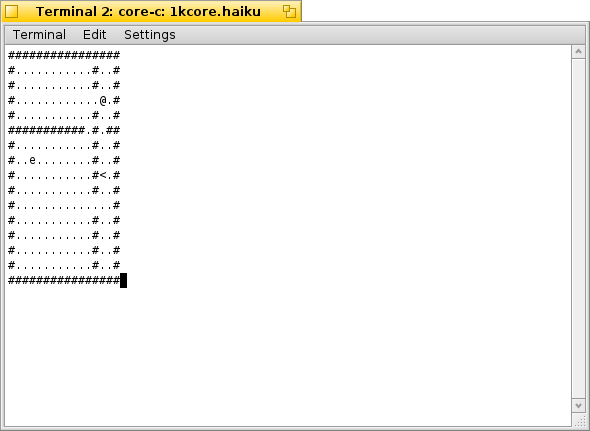# coreRL in 1kib

the importance of whitespace

CoreRL is a minimal roguelike with the barest of features: procedural levels, increasing difficulty, a basic AI, and a level-based score. Originally requiring 4KiB of C-source code, this revised version maintains all the existing features and error detection packed into just under 1KiB of source code:

#include <curses.h> #include <stdlib.h> #define V(a,b) rand()%(b-(a))+a #define L(a,b,c) for(a=b;a<c;++a) #define M m[r][c] #define E 16 #define f 46 #define w 35 int l,R[E],C[E];main(){int i,r,c,k,d;char *s,m[E][E]; initscr();cbreak();srand(time(0));keypad(stdscr,1);A: L(r,0,E)L(c,0,E)M=r&&r<15&&c&&c<15?f:w;r=V(2,13);c= V(2,13);L(i,1,E)m[r][i]=m[i][c]=w;m[r][V(1,c)]=m[r] [V(c+1,14)]=m[V(1,r)][c]=m[V(r+1,14)][c]=f;while(m[r= V(1,E)][c=V(1,E)]^f);M=60;++l;L(i,0,(l<E?l+1:E)){while (r=V(1,E),c=V(1,E),M^f);R[i]=r;C[i]=c;M=i?'e':64;}while (1)L(i,0,E){r=R[i];c=C[i];if(i){if(!r)goto B;d=(*R-r>0) -(*R-r<0);k=m[r+d][c];r+=k^64&&k^f?0:d;k=R[i]^r?0:(*C -c>0)-(*C-c<0);d=m[r][c+k];c+=d^64&&d^f?0:k;if(s="Died" ,M==64)goto Q;}else{L(k,0,E)mvaddnstr(k,0,m[k],E);k= getch();k^258||++r;k^259||--r;k^260||--c;k^261||++c;if (s="Quit",k=='q')goto Q;if(M==60)goto A;if(M^'e'&&M^f) break;if(M^f)L(k,0,E)if(R[k]==r&&C[k]==c){M=f;R[k]=0; goto B;}}M^f||(M=i?'e':64,m[R[i]][C[i]]=f,R[i]=r,C[i] =c);B:;}Q:endwin();printf("%s on level %d.\n",s,l); exit(0);}Level 1. Our protagonist `@` is in the upper-right room, with `e`vil in the lower-left and the stairs `<` in the lower-right.

Use the arrow keys to move and q to quit. Bumping into enemies will remove them, and moving onto the stairs will advance to the next level.

In additon to the tricks described for Monster Caves, shrinking CoreRL to 1023 bytes required implicitly defining main's return type and using the comma operator in conditionals. As the comma operator evaluates to its rightmost value, conditionals such as `if(s="Died",M==64)` reduce the total number of curly braces.

Originally, it also included macros for infinite loops and integer declarations. Reducing the used variables and rewriting loops to be finite enabled those definitions to be removed. And unlike Monster Caves, including curses is necessary since CoreRL uses the arrow keys (and therefore refers to `stdscr`) instead of the numeric keypad.

This version of the source is also released into the public domain and was developed on Haiku alpha 4.1 (binary). It was tested on OS X 10.8.5 (binary) and Debian Linux (thanks to nandryshak). MEaster has also ported it to DCPU-16 assembly (source; binary). Compile on systems with ncurses and gcc using:

gcc -o corerl 1kcore.c -lncurses

An annotated version of the source follows:

// We need curses because we're using the arrow keys; // Vi-keys would enable us to omit this. ncurses.h // just includes curses.h, so this saves a byte. #include <curses.h> #include <stdlib.h> // Generates a random Value in [a, b). #define V(a,b) rand()%(b-(a))+a // Our Loop macro. #define L(a,b,c) for(a=b;a<c;++a) // Access the map. #define M m[r][c] // The maximum number of Entities; also used as // the map size. #define E 16 // ASCII . #define f 46 // ASCII # #define w 35 // The level and entities' Rows and Columns. int l,R[E],C[E]; // Default return type is int. main(){ // index, row, column, and two scratch variables. int i,r,c,k,d; // The exit string and map. char *s,m[E][E]; // Readies ncurses for immediate input using // the arrow-keys & seeds the random number // generator. initscr(); cbreak(); srand(time(0)); keypad(stdscr,1); // New level. A: // Walls on the edge, floor inside. L(r,0,E)L(c,0,E)M=r&&r<15&&c&&c<15?f:w; // Generates the wall intersection point. r=V(2,13); c=V(2,13); // Draws the interior walls. L(i,1,E)m[r][i]=m[i][c]=w; // Add the floor between rooms. m[r][V(1,c)]=m[r][V(c+1,14)] =m[V(1,r)][c]=m[V(r+1,14)][c]=f; // Loops until we have a floor // at m[r][c], and then puts the // stairs there. while(m[r=V(1,E)][c=V(1,E)]^f); M=60; // Use a 1-based level for exit messages. ++l; // Place the player and l enemies. L(i,0,(l<E?l+1:E)){ while(r=V(1,E),c=V(1,E),M^f); R[i]=r; C[i]=c; M=i?'e':64; } // The main loop. while(1) // For each entity, L(i,0,E){ // Grab its current location. r=R[i]; c=C[i]; // For enemies, if(i){ // Is it inactive? if(!r)goto B; // Our basic AI: Move towards // the player first by row // and then by column. d=(*R-r>0)-(*R-r<0); k=m[r+d][c]; r+=k^64&&k^f?0:d; // Only tries moving horizontally // if we didn't move vertically. k=R[i]^r?0:(*C-c>0)-(*C-c<0); d=m[r][c+k]; c+=d^64&&d^f?0:k; // Did an entity eat the player? if(s="Died",M==64)goto Q; } // For the player, entity 0, else{ // Redraw the map. L(k,0,E)mvaddnstr(k,0,m[k],E); // If k is the target key, k^value // is 0, so we need to change the // target space. k=getch(); k^258||++r; k^259||--r; k^260||--c; k^261||++c; // Are they quitting? if(s="Quit",k=='q')goto Q; // Enter the stairs? Next level. if(M==60)goto A; // If the player isn't moving onto // an enemy or a floor space by this // point, then force them to move. if(M^'e'&&M^f)break; // If the target's not a floor tile, // it must be another entity. if(M^f) // Remove the offending entity. Oddly // enough, the while(invalid); loop // trick used elsewhere of this line // requires the same number of bytes. L(k,0,E)if(R[k]==r&&C[k]==c){ M=f; R[k]=0; goto B; } } // Move the current entity if we can. M^f||(M=i?'e':64,m[R[i]][C[i]]=f,R[i]=r,C[i]=c); // The target for continue-equivalent gotos. // The semicolon is required for compiling on OS X. B:; } // We're done: Cleanup and display what happened. Q: endwin(); printf("%s on level %d.\n",s,l); exit(0); }